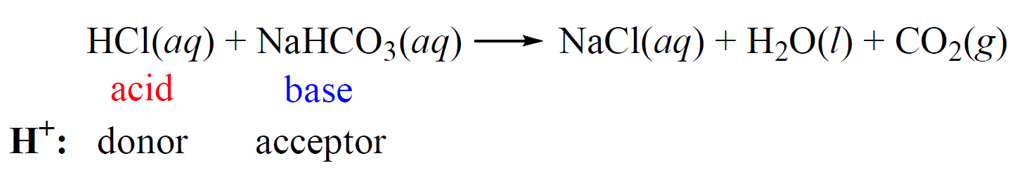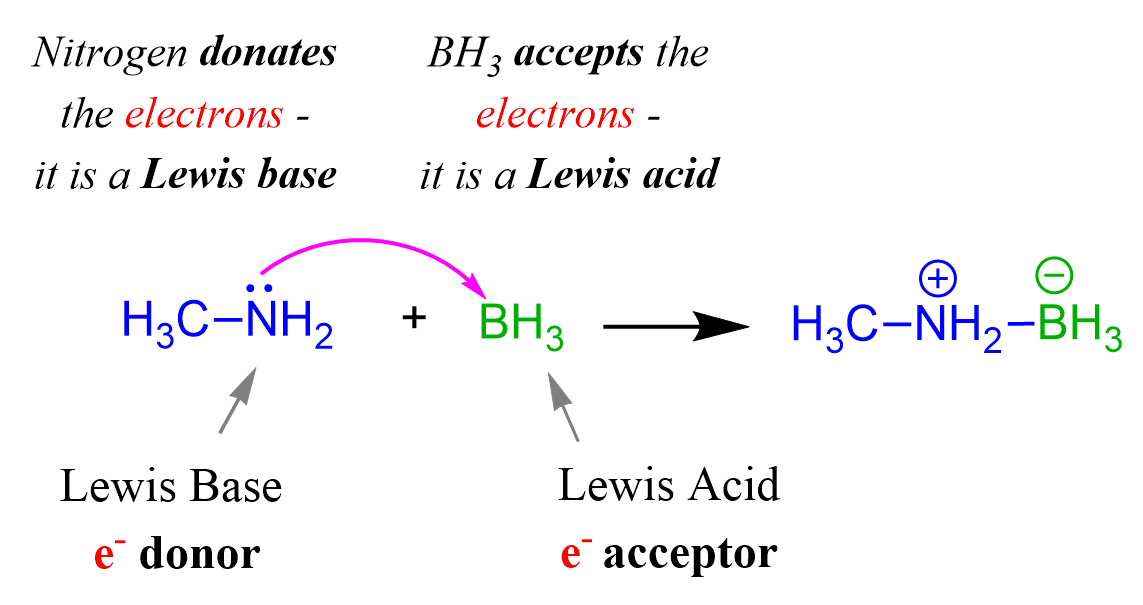## General Chemistry

There are three theories for classifying acids and bases.

# The Arrhenius Theory of Acids and Bases

This is the oldest acid-base theory proposed by Swedish chemist Svante Arrhenius in 1884.

According to the Arrhenius acid-base theory, acids are compounds that dissociate in water forming protons (H+) and bases dissociate in water to form a hydroxide ion (OH).

For examples,

An acid:    HCl(aq) → H+(aq) + Cl(aq)

A base:    NaOH(aq) → Na+(aq) + OH(aq)

# The Brønsted–Lowry Acid–Base Theory

The limitation of the Arrhenius theory is that it classifies only hydroxides are bases and while it is an accurate characteristic for them, there are other species that can fall into this category as well.

For example, hydrochloric acid readily reacts with baking soda producing NaCl, carbon dioxide (CO2) and water.

HCl(aq) + NaHCO3(aq) → NaCl(aq) + H2O(l) + CO2(g)

Now, it is known that acid-base reactions are one of the fastest and therefore, another theory is needed to classify sodium bicarbonate (NaHCO3) as a base.

And that theory is the Brønsted–Lowry acid-base theory which states that acids are proton donors, and bases are proton acceptors. Sodium bicarbonate and many other compounds classify as bases according to this theory even though they do not contain a hydroxide ion.Another example showing the broader definition of bases by the Brønsted–Lowry theory is, for example, the reaction of ammonia (NH3) with HCl forming ammonium chloride:

NH3       +      HCl              NH4 Cl
base              acid                 salt

Ammonia is the proton acceptor and therefore, the base, while HCl is the proton donor, so it is the acid.

# Lewis Acid-Base Theory

Gilbert Newton Lewis (same Lewis who is behind the electron-dot formulas) suggested even a more general way of classifying acids and bases.

A Lewis acid is an electron pair acceptor.

A Lewis base is an electron pair donor.

What is different compared with the Arrhenius and Brønsted approaches is that Lewis took the opposite view and instead of focusing at the proton, he looked from the perspective of electrons

So, how is this better than the Brønsted theory?

Just like the Brønsted theory is broader and more universal than the Arrhenius theory, the Lewis acid-base theory can also classify more (in fact almost any) compound as a Lewis acid or a base.

For example, methylamine (CH3NH2) is a derivative of ammonia, and it acts as base when reacted with an acid by donating its lone pair of electrons of the nitrogen:The HCl is the acid because; 1) it accepts the electrons from the nitrogen, 2) it is a proton donor, so it is still a Brønsted acid.

Now, if we replace the HCl with borane (BH3), we can neither classify it as an acid or a base by the Arrhenius, nor by the Brønsted theory because there is no proton involved in the reaction:

CH3NH2      +      BH3              (CH3NH2)+-(BH3)
base               acid                           salt

What is happening is that the nitrogen donates in lone pair of electrons to boron which can accept it because of an empty orbital.The acid and base in this reaction can only be classified based on the electron transfer between them, and therefore, we can only identify the acid and the base by the Lewis theory.

So, to summarize,

• The Arrhenius theory is the oldest acid-base theory which is still great if the base is a hydroxide.
• The Brønsted-Lowry theory focuses on the proton only; the proton donor is the acid, and the proton acceptor is the base.
• The Lewis acid-base theory is the most universal as it can classify the acid and the base in any ionic reaction. There is a more detailed post about the Lewis acid-base theory tailored more toward organic chemistry which you can find here.

I know that the Lewis acid-base reactions are unusual at this point and may have caused some confusion. The good news is that it is not used in general chemistry as often, and therefore, you can still visualize acids as compounds having an H in the beginning and bases having an OH keeping in mind the other definitions when needed.

HCl is an acid, NaOH is a base – what a great reference to recognize acids and bases!

Check Also